Скачать презентацию Approximation Algorithms for Non-Uniform Buy-at-Bulk Network Design Problems

51a75716159298372f28a1c9df64335a.ppt

• Количество слайдов: 30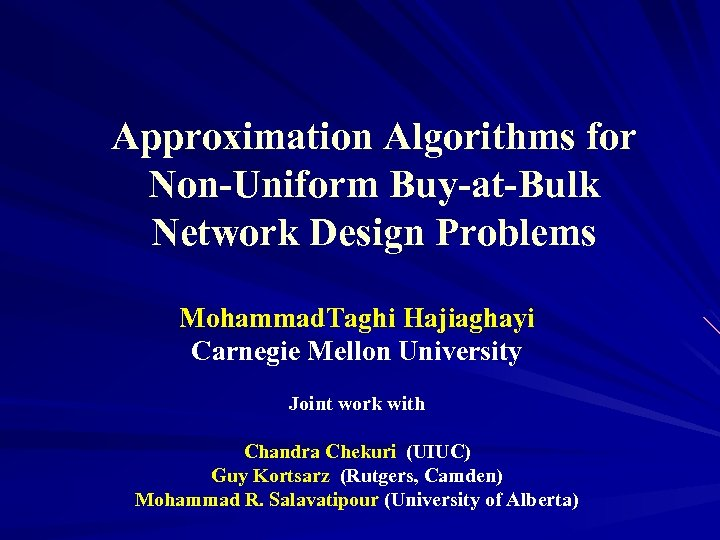Approximation Algorithms for Non-Uniform Buy-at-Bulk Network Design Problems Mohammad. Taghi Hajiaghayi Carnegie Mellon University Joint work with Chandra Chekuri (UIUC) Guy Kortsarz (Rutgers, Camden) Mohammad R. Salavatipour (University of Alberta)Motivation Suppose we are given a network and some nodes have to be connected by cables Each cable has a cost (installation or cost of usage) Question: Install cables satisfying demands at minimum cost 5 3 14 8 21 9 10 11 16 21 7 27 12 This is the well-studied Steiner forest problem and is NP-hard 2Motivation (cont’d) Consider buying bandwidth to meet demands between pairs of nodes. The cost of buying bandwidth satisfy economies of scale The capacity on a link can be purchased at discrete units: Costs will be: Where 3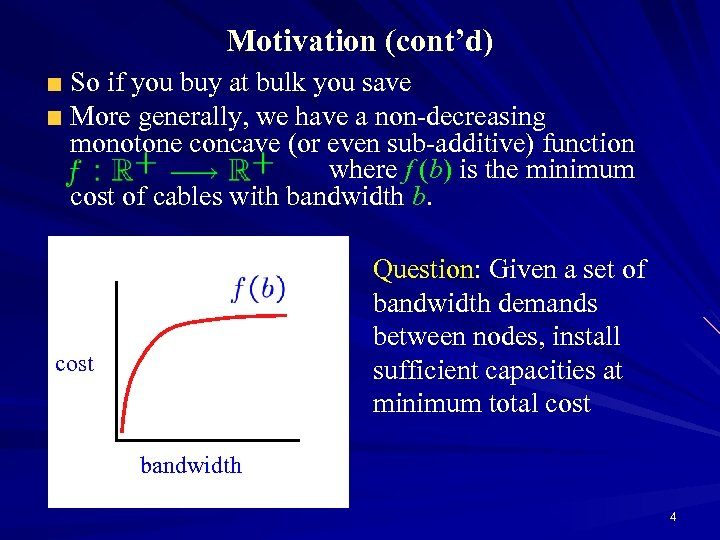Motivation (cont’d) So if you buy at bulk you save More generally, we have a non-decreasing monotone concave (or even sub-additive) function where f (b) is the minimum cost of cables with bandwidth b. Question: Given a set of bandwidth demands between nodes, install sufficient capacities at minimum total cost bandwidth 4Motivation (cont’d) The previous problem is equivalent to the following problem: There a set of pairs to be connected For each possible cable connection e we can: Buy it at b(e): and have unlimited use Rent it at r(e): and pay for each unit of flow A feasible solution: buy and/or rent some edges to connect every si to ti. Goal: minimize the total cost 5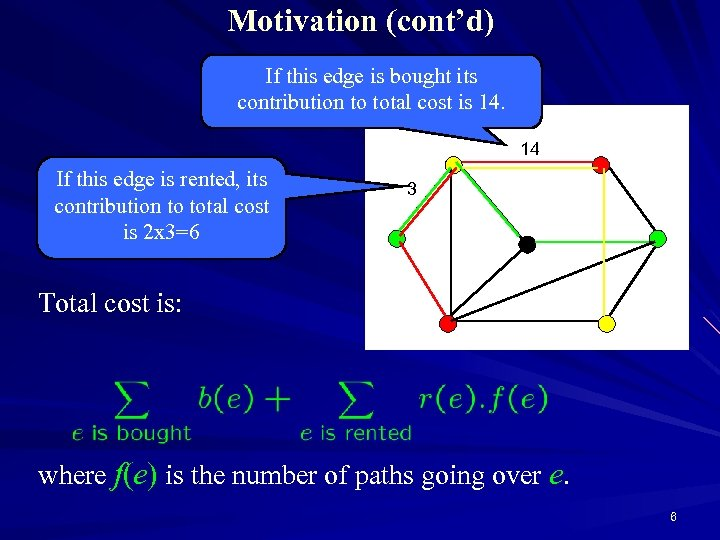Motivation (cont’d) If this edge is bought its contribution to total cost is 14. 14 If this edge is rented, its contribution to total cost is 2 x 3=6 3 10 Total cost is: where f(e) is the number of paths going over e. 6Cost-Distance These problems are also known as cost-distance problems: cost function length function Also a set of pairs of nodes each with a demand for every i Feasible solution: a set s. t. all pairs are connected in 7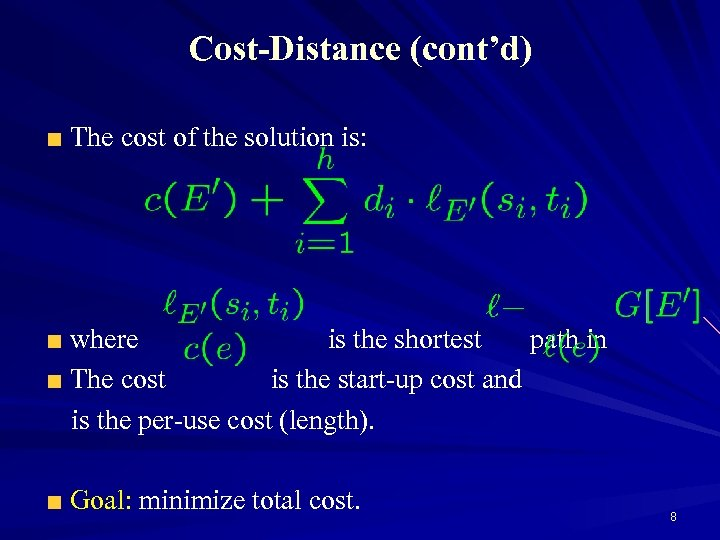Cost-Distance (cont’d) The cost of the solution is: where is the shortest path in The cost is the start-up cost and is the per-use cost (length). Goal: minimize total cost. 8Multicommodity Buy At Bulk The problem is called Multi-Commodity Buy-at-Bulk (MC-BB) Note that the solution may have cycles 5 11 8 12 21 9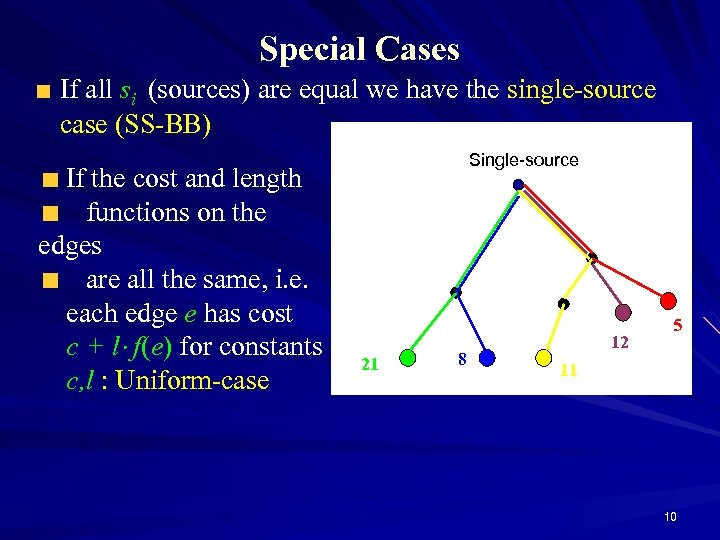Special Cases If all si (sources) are equal we have the single-source case (SS-BB) If the cost and length functions on the edges are all the same, i. e. each edge e has cost c + l f(e) for constants c, l : Uniform-case Single-source 21 8 12 5 11 10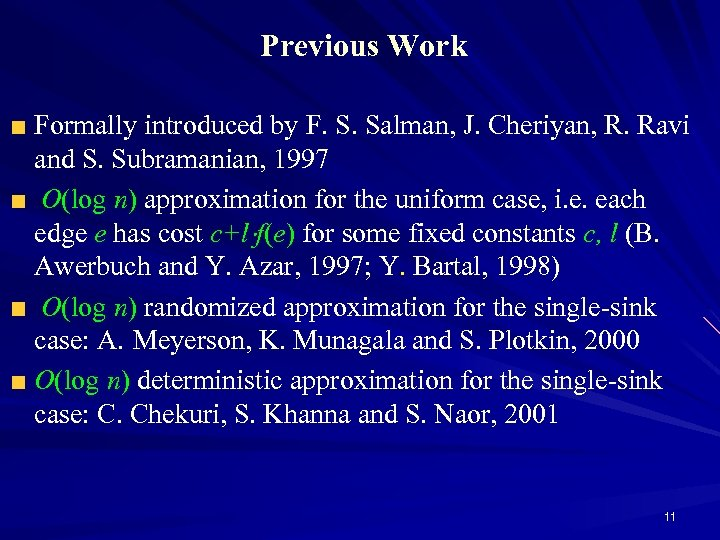Previous Work Formally introduced by F. S. Salman, J. Cheriyan, R. Ravi and S. Subramanian, 1997 O(log n) approximation for the uniform case, i. e. each edge e has cost c+l f(e) for some fixed constants c, l (B. Awerbuch and Y. Azar, 1997; Y. Bartal, 1998) O(log n) randomized approximation for the single-sink case: A. Meyerson, K. Munagala and S. Plotkin, 2000 O(log n) deterministic approximation for the single-sink case: C. Chekuri, S. Khanna and S. Naor, 2001 11Hardness Results for Buy-at-Bulk Problems Hardness of Ω(log n) for the singlesink case J. Chuzhoy, A. Gupta, J. Naor and A. Sinha, 2005 Ω(log 1/2 - n) in general M. Andrews 2004, unless NP ZPTIME(npolylog(n)) 12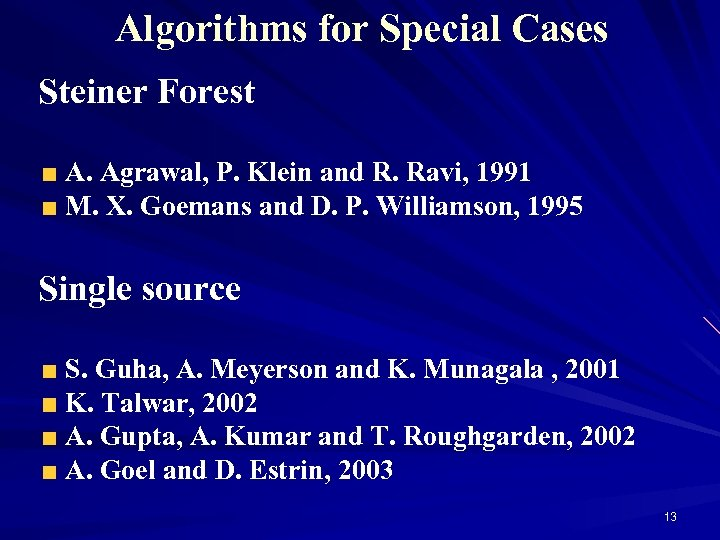Algorithms for Special Cases Steiner Forest A. Agrawal, P. Klein and R. Ravi, 1991 M. X. Goemans and D. P. Williamson, 1995 Single source S. Guha, A. Meyerson and K. Munagala , 2001 K. Talwar, 2002 A. Gupta, A. Kumar and T. Roughgarden, 2002 A. Goel and D. Estrin, 2003 13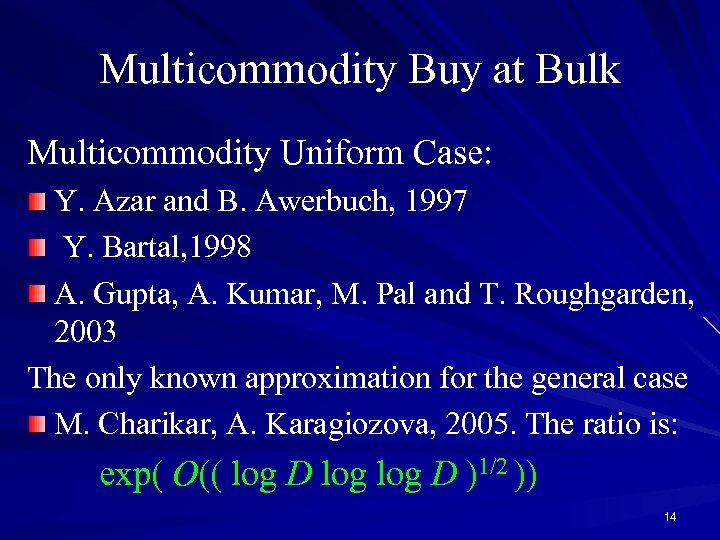Multicommodity Buy at Bulk Multicommodity Uniform Case: Y. Azar and B. Awerbuch, 1997 Y. Bartal, 1998 A. Gupta, A. Kumar, M. Pal and T. Roughgarden, 2003 The only known approximation for the general case M. Charikar, A. Karagiozova, 2005. The ratio is: exp( O(( log D )1/2 )) 14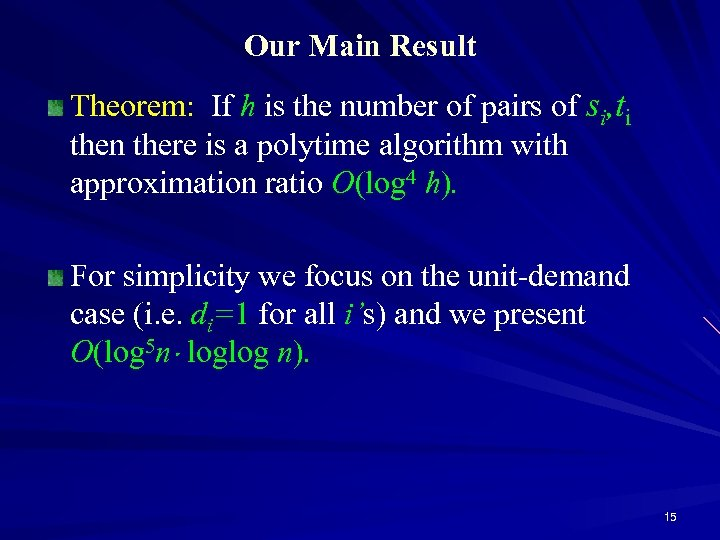Our Main Result Theorem: If h is the number of pairs of si, ti then there is a polytime algorithm with approximation ratio O(log 4 h). For simplicity we focus on the unit-demand case (i. e. di=1 for all i’s) and we present O(log 5 n loglog n). 15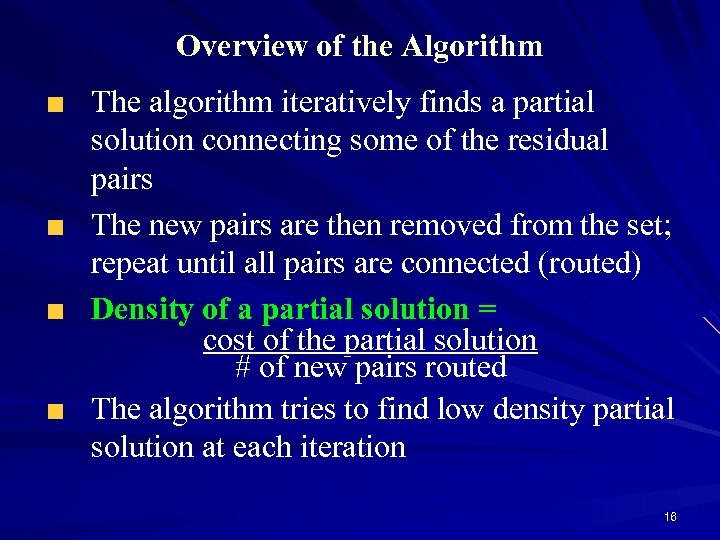Overview of the Algorithm The algorithm iteratively finds a partial solution connecting some of the residual pairs The new pairs are then removed from the set; repeat until all pairs are connected (routed) Density of a partial solution = cost of the partial solution # of new pairs routed The algorithm tries to find low density partial solution at each iteration 16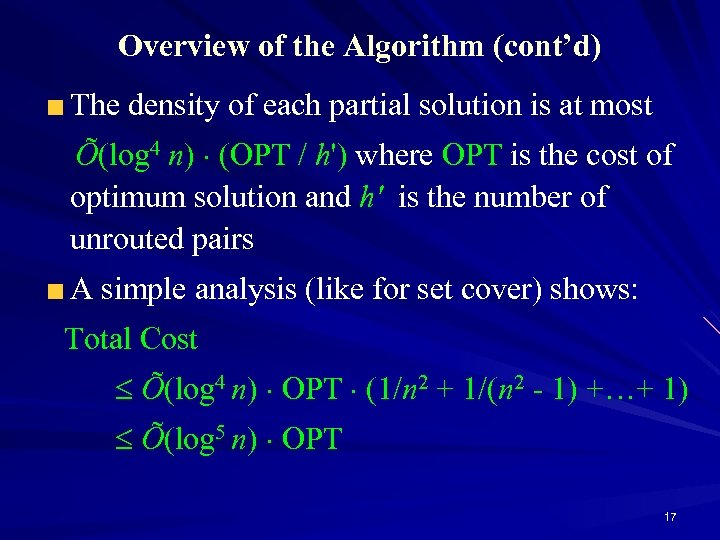Overview of the Algorithm (cont’d) The density of each partial solution is at most Õ(log 4 n) (OPT / h') where OPT is the cost of optimum solution and h' is the number of unrouted pairs A simple analysis (like for set cover) shows: Total Cost Õ(log 4 n) OPT (1/n 2 + 1/(n 2 - 1) +…+ 1) Õ(log 5 n) OPT 17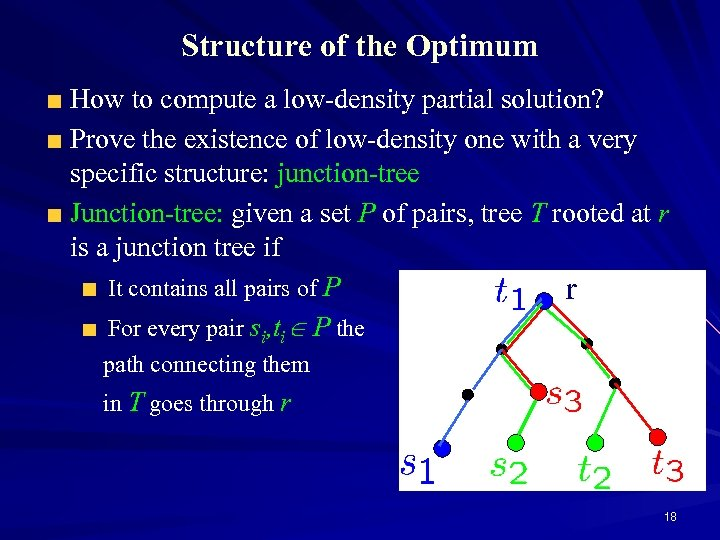Structure of the Optimum How to compute a low-density partial solution? Prove the existence of low-density one with a very specific structure: junction-tree Junction-tree: given a set P of pairs, tree T rooted at r is a junction tree if It contains all pairs of P r For every pair si, ti P the path connecting them in T goes through r 18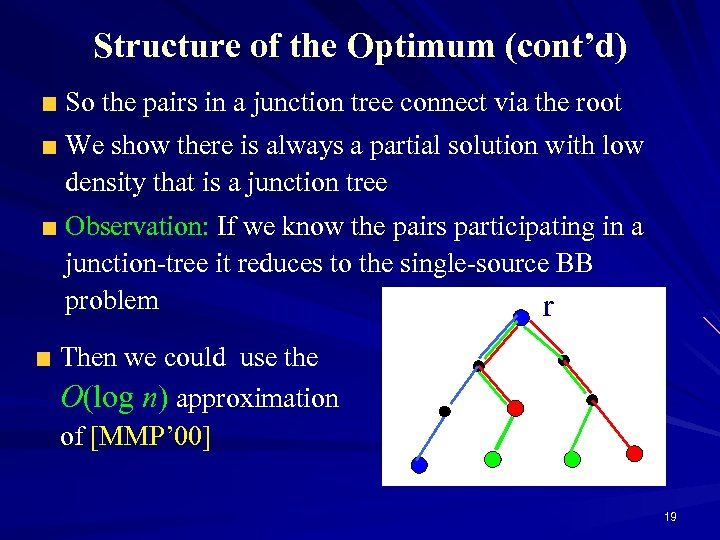Structure of the Optimum (cont’d) So the pairs in a junction tree connect via the root We show there is always a partial solution with low density that is a junction tree Observation: If we know the pairs participating in a junction-tree it reduces to the single-source BB problem r Then we could use the O(log n) approximation of [MMP’ 00] 19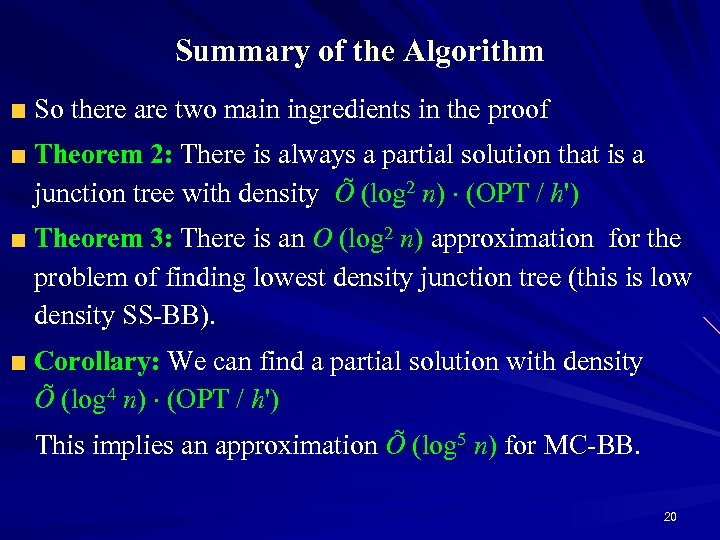Summary of the Algorithm So there are two main ingredients in the proof Theorem 2: There is always a partial solution that is a junction tree with density Õ (log 2 n) (OPT / h') Theorem 3: There is an O (log 2 n) approximation for the problem of finding lowest density junction tree (this is low density SS-BB). Corollary: We can find a partial solution with density Õ (log 4 n) (OPT / h') This implies an approximation Õ (log 5 n) for MC-BB. 20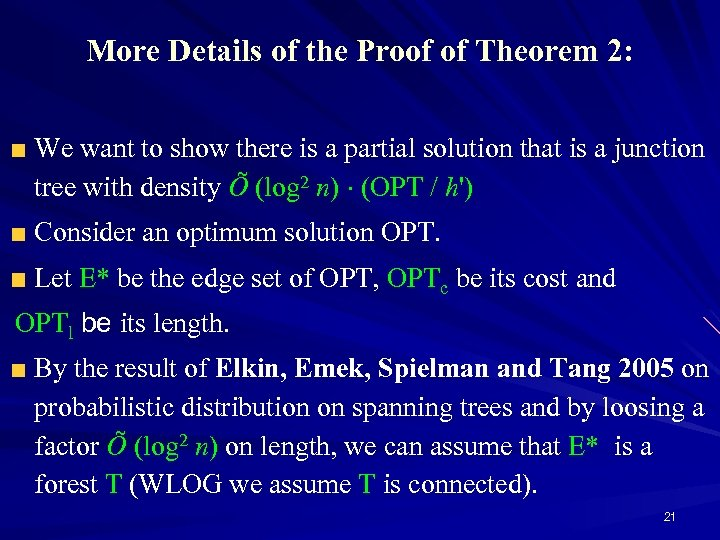More Details of the Proof of Theorem 2: We want to show there is a partial solution that is a junction tree with density Õ (log 2 n) (OPT / h') Consider an optimum solution OPT. Let E* be the edge set of OPT, OPTc be its cost and OPTl be its length. By the result of Elkin, Emek, Spielman and Tang 2005 on probabilistic distribution on spanning trees and by loosing a factor Õ (log 2 n) on length, we can assume that E* is a forest T (WLOG we assume T is connected). 21More Details of the Proof of Theorem 2: From T we obtain a collection of rooted subtrees T 1, …, Ta such that any edge e of T is in at most O(log h) of the subtrees For every pair there is exactly one index i such that both vertices are in Ti; further the root of Ti is their least common ancestor The total cost of the junction trees is at most Õ (log 2 h) OPT (O (log h) OPTc + Õ (log 2 h) OPTl) Thus at least one of junction trees of T 1, …, Ta has the desired density of Õ (log 2 h) (OPT / h') 22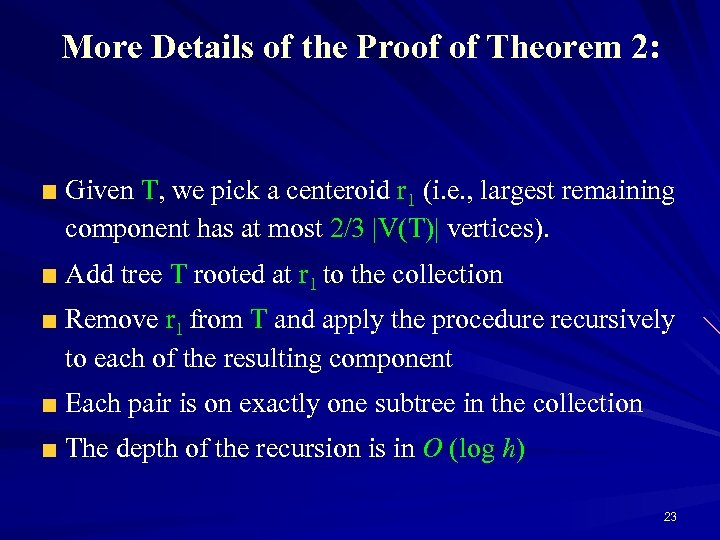More Details of the Proof of Theorem 2: Given T, we pick a centeroid r 1 (i. e. , largest remaining component has at most 2/3 |V(T)| vertices). Add tree T rooted at r 1 to the collection Remove r 1 from T and apply the procedure recursively to each of the resulting component Each pair is on exactly one subtree in the collection The depth of the recursion is in O (log h) 23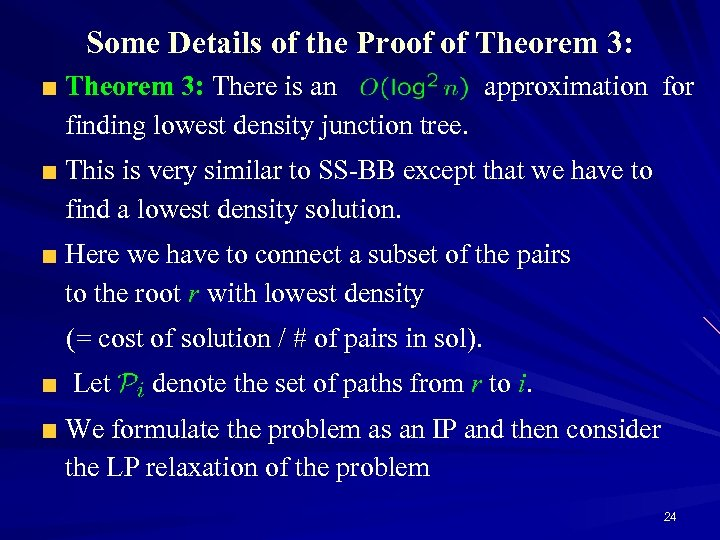Some Details of the Proof of Theorem 3: There is an approximation for finding lowest density junction tree. This is very similar to SS-BB except that we have to find a lowest density solution. Here we have to connect a subset of the pairs to the root r with lowest density (= cost of solution / # of pairs in sol). Let denote the set of paths from r to i. We formulate the problem as an IP and then consider the LP relaxation of the problem 24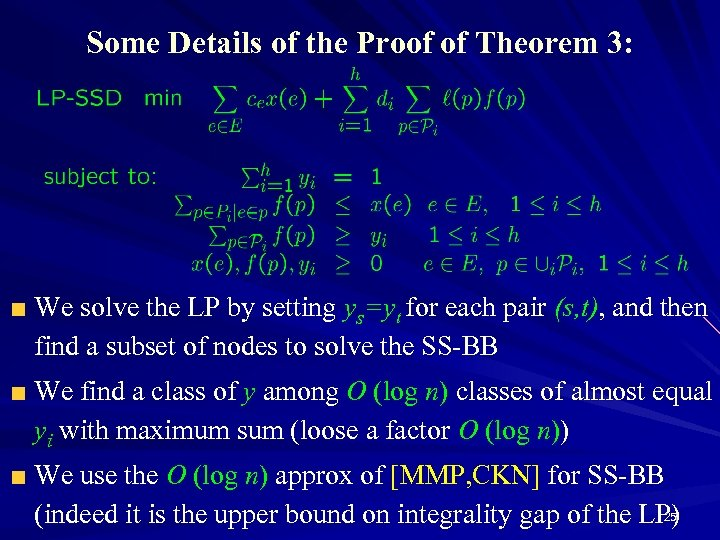Some Details of the Proof of Theorem 3: We solve the LP by setting ys=yt for each pair (s, t), and then find a subset of nodes to solve the SS-BB We find a class of y among O (log n) classes of almost equal yi with maximum sum (loose a factor O (log n)) We use the O (log n) approx of [MMP, CKN] for SS-BB 25 (indeed it is the upper bound on integrality gap of the LP)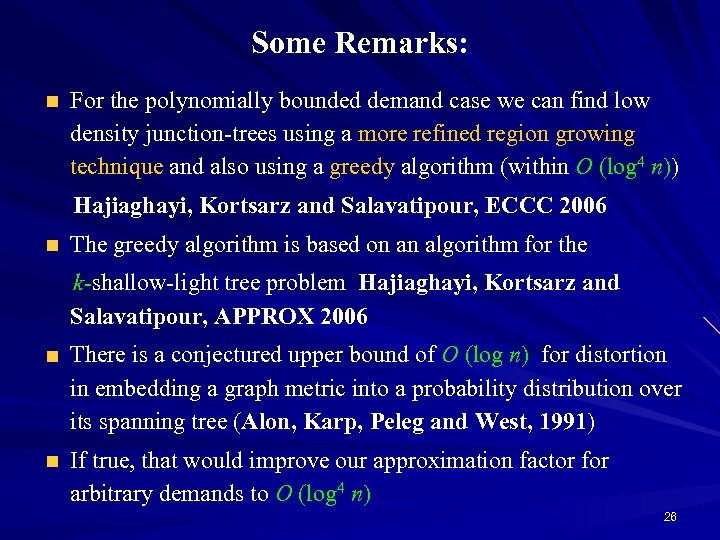Some Remarks: For the polynomially bounded demand case we can find low density junction-trees using a more refined region growing technique and also using a greedy algorithm (within O (log 4 n)) Hajiaghayi, Kortsarz and Salavatipour, ECCC 2006 The greedy algorithm is based on an algorithm for the k-shallow-light tree problem Hajiaghayi, Kortsarz and Salavatipour, APPROX 2006 There is a conjectured upper bound of O (log n) for distortion in embedding a graph metric into a probability distribution over its spanning tree (Alon, Karp, Peleg and West, 1991) If true, that would improve our approximation factor for arbitrary demands to O (log 4 n) 26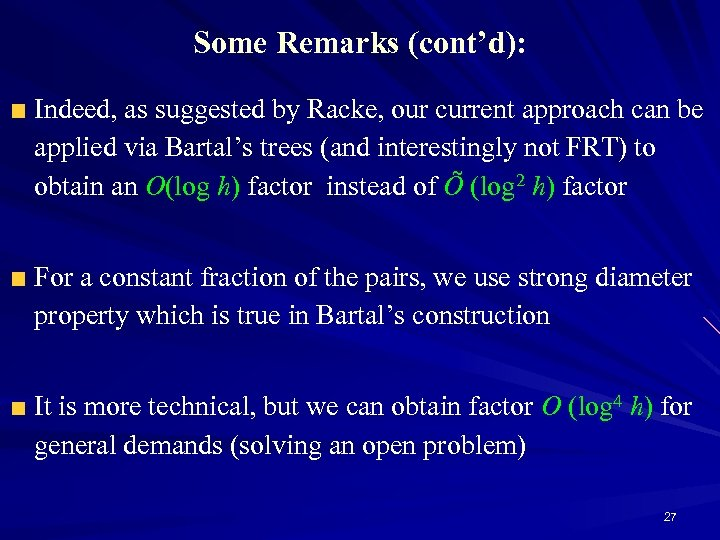Some Remarks (cont’d): Indeed, as suggested by Racke, our current approach can be applied via Bartal’s trees (and interestingly not FRT) to obtain an O(log h) factor instead of Õ (log 2 h) factor For a constant fraction of the pairs, we use strong diameter property which is true in Bartal’s construction It is more technical, but we can obtain factor O (log 4 h) for general demands (solving an open problem) 27Recent Extensions The result O(log 4 n) can be extended to the vertex-weighted case but requires some new ideas and some extra work [CHKS’ 07]. Especially we obtain the tight result O(log n) for the single-sink vertex-weighted case via LP rounding Also it needs some subtle change of vertex weights to edge weights in the junction tree lemma Also our results can be extended to the stochastic versions with non-uniform inflation (by loosing an extra factor O(log n)) [Gupta, Hajiaghayi, Kumar’ 06]. Some technique has been used in the Dial-a-Ride problem [Gupta, Hajiaghayi, Ravi, Nagarajan’ 06]. 28Open Problems There are still quite large gaps between upper bounds (approx alg) and lower bounds (hardness) For MC-BB: vs For SS-BB: vs It would be nice to upper bound the integrality gap for MC-BB. Emphasize on the conjecture of Alon, Karp, Peleg and West, 1991 29Thanks for your attention… 30